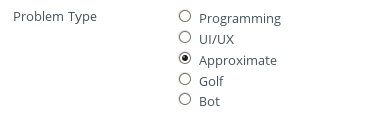# Problem Setter Guide

### General Problem Setting Guide

Problem setters at HackerEarth have access to a special dashboard for adding the problems. This also helps them in testing their problems directly on the online judge. You are not supposed to email the problems.

For each problem you submit, you must provide

• Problem statement: It must contain the description of the problem, format of input and output and the constraints on input each in a separate section.
• Input and output files: Set of Input test case files and corresponding output files that are used to judge the solution.
• Difficulty level: Difficulty level of the problem which is to be agreed upon by both the setter and tester.
• Solution: Actual solution to the problem that is well commented.
• Editorial: An editorial describing the expected algorithm to solve the problem.
• Tags: Tags to be entered for each problem. These tags must be combination of following:  One of these:
• Easy
• Medium
• Hard

Any combination of these: - Algorithms - Basic-Programming - Data-Structures - Math

You can put any other relevant tag you might want too. But the tags mentioned above must be there.  Valid example of tags for a problem is: Easy, Algorithms, Data-Sructures, String-Manipulation

General guidelines for writing problem statement.

• Problem statement must be clear with no ambiguity so that everyone interprets in the same way.
• It should clearly specify the format of input and output along with the constraints.
• It must be be long enough to be clear and short enough to be interesting.
• Problem statement must be properly formatted. Variables, formulae, relevant text must be put in bold. Check a few examples where all these rules are followed: Counting StringsGame of survival
• It must be grammatically correct.

General guidelines for choosing problems.

• It must not be taken for other sites.
• Solution must not be available easily on the internet.
• It must not be a standard problem. (Easy/Cakewalk problems can be standard problems.)

General guidelines for setting the problem:

• Elaborate the problem statement or build a nice story on it. It must not ask user to implement the algorithm directly. The final problem statement must be interesting and engage users to think and solve the problem.
• You can add as many test case files as you can. It must cover all corner cases to make sure that the solution that passes the test files is correct.
• General tip is to have easy test cases, the cases that have lower constraints, that are not corner cases in few of the test files. This is because a user who cannot solve the problem completely or writes a correct but naive solution, gets the partial credit on the easy ones.
• Few of the test files should have input cases of maximum limit to make sure a naive solution exceeds the time limit on those input files.

Check out techniques and tricks used in problem setting.

### Approximate Problem Setting Guide

An approximate problem may be maximization or minimization type.

Following things are needed for an approximate problem: (Before you’re allowed to save the problem!)

Make sure you don’t miss out on doing this:• Problem Statement
• Scoring Procedure
• Sample Input
• Sample Output
• Sample I/O explaination
• Constraints
• Test Files
• Add at least 10 input files
• No output file (Because correctness of solution will be determined by checker)
• Checker (in any language i.e. c/cpp/java/python etc.)
( Without a checker, you cannot save an approximate problem! )

Checker Format:
The checker basically checks the correctness of a solution on the input file and calculates the score of output, if the solution is correct. Since, the checker is evaluated for each input file so there will be some score for each input file and total score will be sum of scores of all input files.

Sample Checker Files:
- Sample Python Checker: https://code.hackerearth.com/cdf6f4k

• Input for checker is Input Test File data followed by the Output of User's solution. Both of them will be available to checker file as STDIN.
• First, read input from Input Test File data (on which output has to be checked)
• Then, read the output produced by User's solution as checker's input.
• Use assertions to check correctness. (in case output is not in required format).
• If some assertion fails it will cause "Wrng Answer" verdict. In java, you might need to throw an exception.
• Use the formula (as specified in the problem) on output data to calculate user score.
• Finally, print score that you want to show for that input file.

### CodeGolf Problem Setting Guide

A Code golf problem is one which participant try to solve using shortest possible code. i.e. smaller the code length more points you will get.

Following things are needed for a codegolf problem.

• problem statement
• sample input
• sample output
• Sample explaination
• constraints
• Test files
• there can be several inputs/output files
• checker that will calculate score based on source length (in any language i.e c/cpp/java/python etc.)
• Formula to calculate score from source length

Checker Format: checker is executed only if submission status is 'AC'. It calculates to score based on source length.

• Input to checker is 'solution file' (yes, you will read this via STDIN)
• Read whole file using STDIN to count total number of characters used(you may include/exclude whitespace according to your requirement)
• Calculate score (out of maximum alloted to problem) using formula described in problem statement.
• Formula: Suppose maximum points are 100, then formula may be (1 - n/max_expected_size)*100. In this case, you can assign zero score to a code which have source length greater than max_ecpected_size. Also Nobody can get 100 points (bcoz source of length zero is not possible) but can only get a score near to 100.

Here you can go through sample checker.

### Apply for Problem Setting/Testing

If you are interested in setting problems for HackerEarth, please fill this form.